# python数据分析——numpy，pandas，matplotlib

matliotilb:帮助画图

numpy：帮助我们处理数据型的数组

pandas：是在numpy的基础上除了帮我们处理数据型的数组，还能帮助我们处理字符串，列表，以及时间序列等等所有数据类型都是可以处理的

1.提出问题

2.准备数据

3.分析数据

4.获得结论

5.成果可视化（图表或者文字等）

jupyter notebook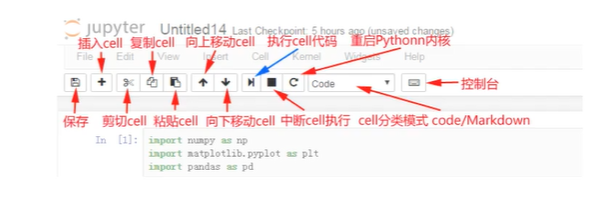matplotlib：最流行的Python底层绘图库，主要做数据可视化图表，名字取材于MATLAB

1.能将数据进行可视化，更直观的呈现

2.使数据更加客观，更具说服力

``````from matplotlib import pyplot as plt
x = range(2,26,2)
y = [15,13,23,24,15,26,22,26,23,11,10,8]#数据在y轴的位置，是一个可迭代对象
#->x轴和y轴的数据一起组成了所有要绘制出的坐标
#->分别是（2，15）（4，13）（6，23（8，24）......

plt.plot(x,y)#->传入x和y，通过plot绘制出折线图
plt.show()#->在执行程序的时候展示图形
``````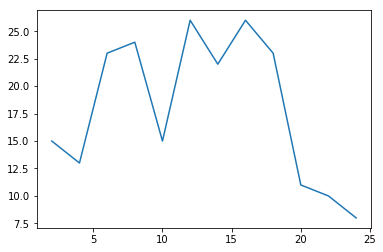``````from matplotlib import pyplot as plt
x = range(2,26,2)
y = [15,13,23,24,15,26,22,26,23,11,10,8]#数据在y轴的位置，是一个可迭代对象
#->x轴和y轴的数据一起组成了所有要绘制出的坐标
#->分别是（2，15）（4，13）（6，23（8，24）......

plt.figure(figsize=(21,10),dpi=100)#设置图片大小
plt.plot(x,y)#->传入x和y，通过plot绘制出折线图
plt.show()#->在执行程序的时候展示图形
``````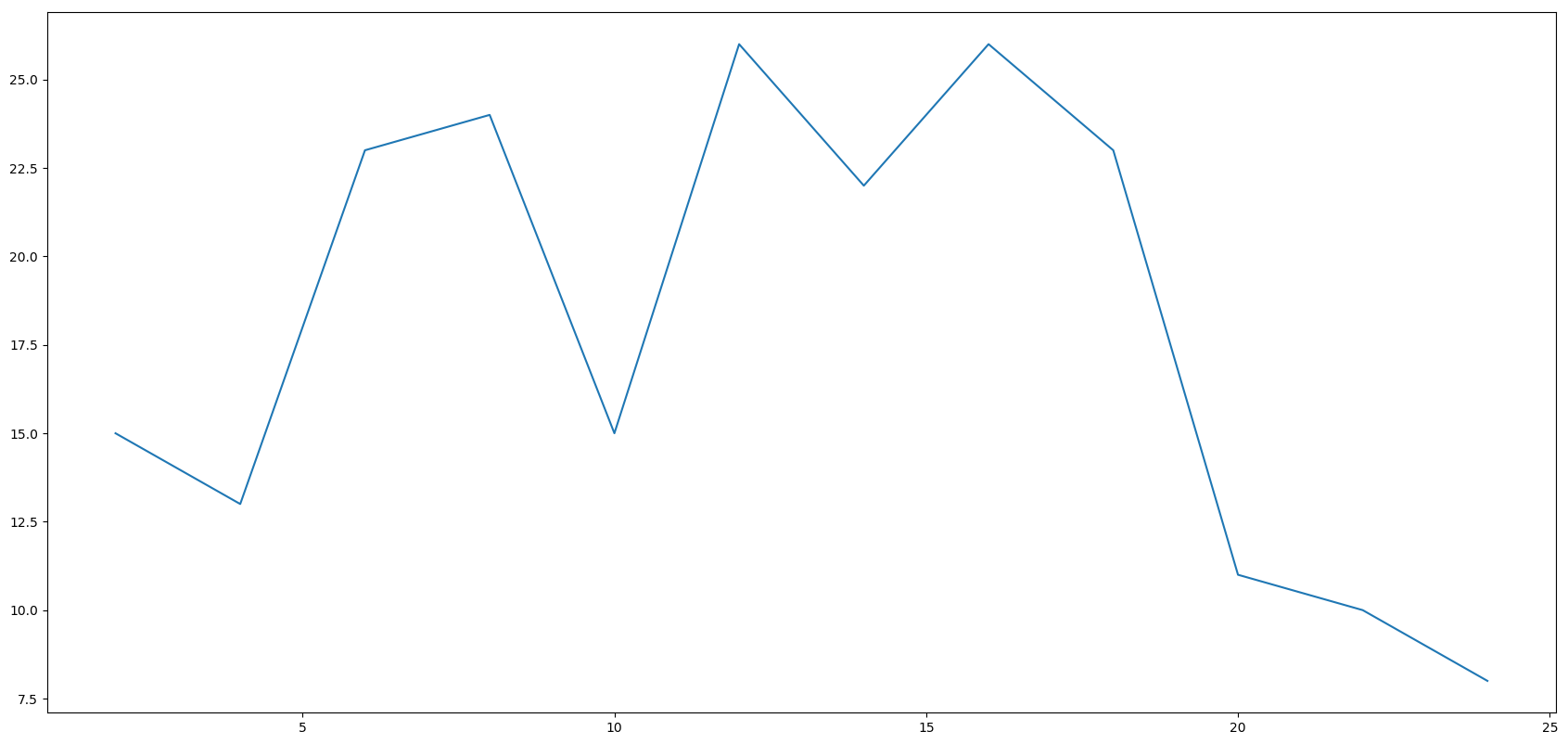``````from matplotlib import pyplot as plt
x = range(2,26,2)
y = [15,13,23,24,15,26,22,26,23,11,10,8]#数据在y轴的位置，是一个可迭代对象
#->x轴和y轴的数据一起组成了所有要绘制出的坐标
#->分别是（2，15）（4，13）（6，23（8，24）......

plt.figure(figsize=(21,10),dpi=100)#设置图片大小
plt.plot(x,y)#->传入x和y，通过plot绘制出折线图
plt.savefig("./sig_size.png")#保存图片，可以保存为svg这种矢量图格式，放大不会有据齿
plt.show()#->在执行程序的时候展示图形
``````plt.xticks(x)

``````from matplotlib import pyplot as plt
x = range(2,26,2)
y = [15,13,23,24,15,26,22,26,23,11,10,8]#数据在y轴的位置，是一个可迭代对象
#->x轴和y轴的数据一起组成了所有要绘制出的坐标
#->分别是（2，15）（4，13）（6，23（8，24）......

plt.figure(figsize=(21,10),dpi=100)#设置图片大小
plt.plot(x,y)#->传入x和y，通过plot绘制出折线图
plt.xticks(x)#绘制x轴的刻度
#plt.savefig("./sig_size.png")#保存图片，可以保存为svg这种矢量图格式，放大不会有据齿
plt.show()#->在执行程序的时候展示图形
``````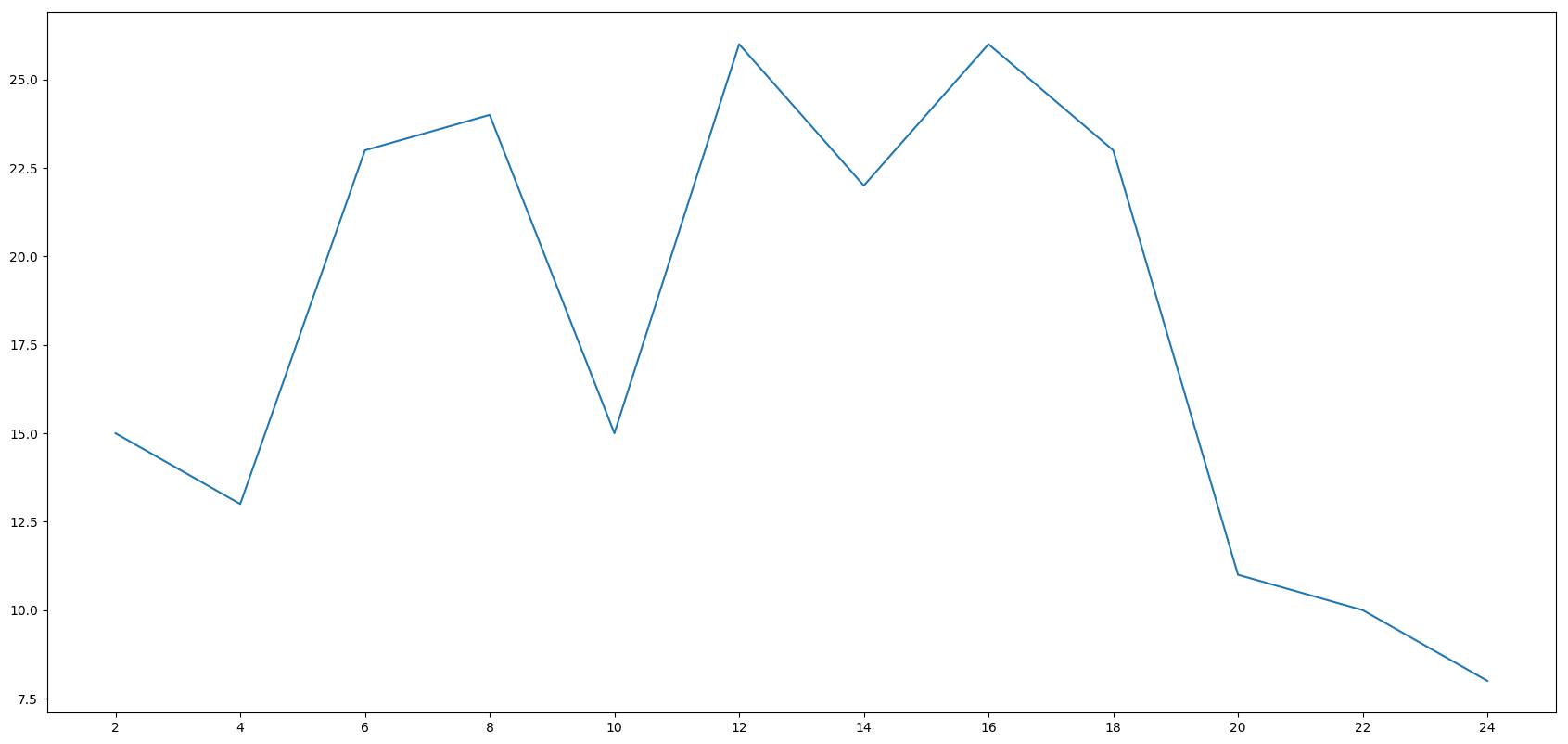plt.xticks(range(2,25,1))#改变范围与步幅

``````from matplotlib import pyplot as plt
x = range(2,26,2)
y = [15,13,23,24,15,26,22,26,23,11,10,8]#数据在y轴的位置，是一个可迭代对象
#->x轴和y轴的数据一起组成了所有要绘制出的坐标
#->分别是（2，15）（4，13）（6，23（8，24）......

plt.figure(figsize=(21,10),dpi=100)#设置图片大小
plt.plot(x,y)#->传入x和y，通过plot绘制出折线图
plt.xticks(range(2,25,1))#绘制x轴的刻度
#plt.savefig("./sig_size.png")#保存图片，可以保存为svg这种矢量图格式，放大不会有据齿
plt.show()#->在执行程序的时候展示图形
``````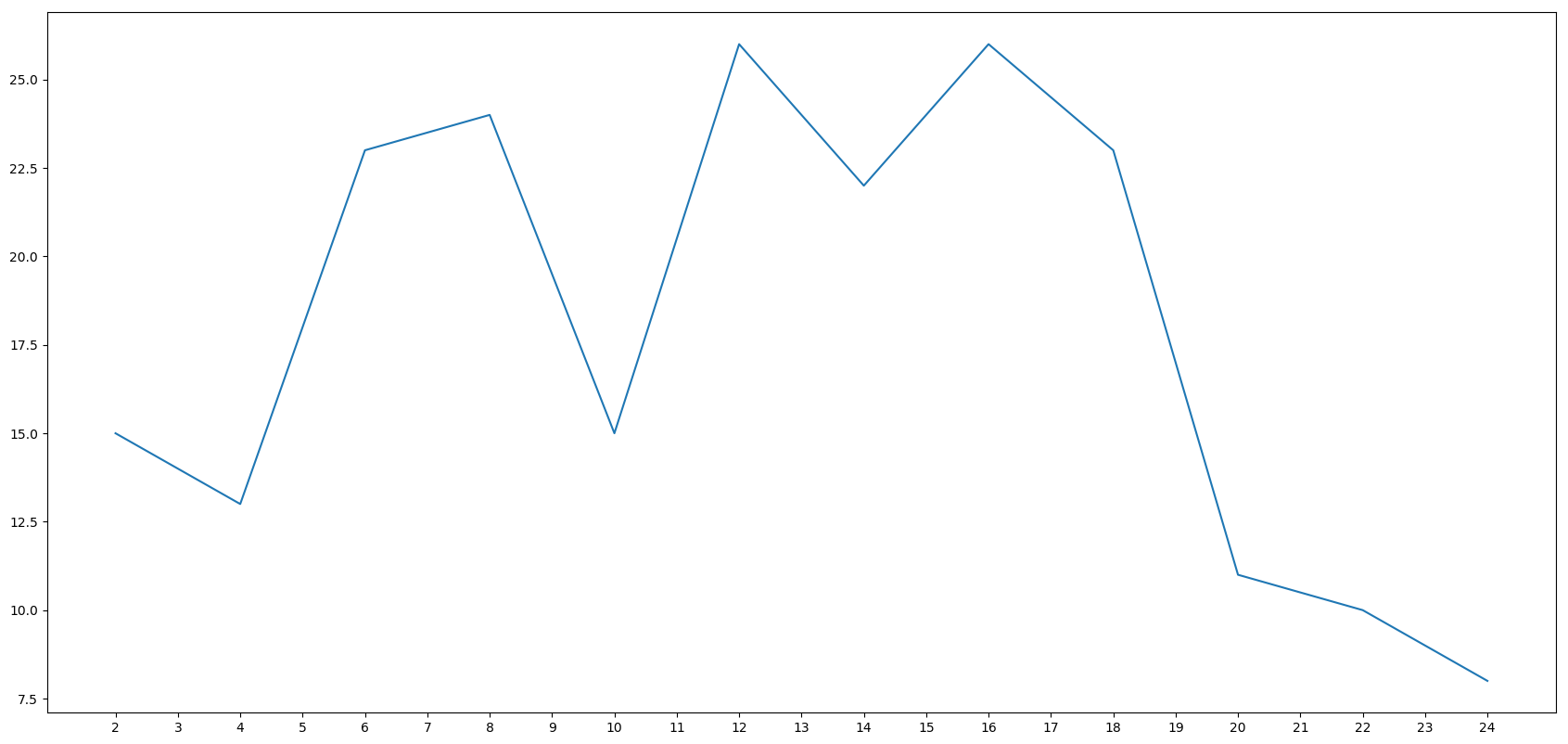``````from matplotlib import pyplot as plt
x = range(2,26,2)
y = [15,13,23,24,15,26,22,26,23,11,10,8]#数据在y轴的位置，是一个可迭代对象
#->x轴和y轴的数据一起组成了所有要绘制出的坐标
#->分别是（2，15）（4，13）（6，23（8，24）......

plt.figure(figsize=(21,10),dpi=100)#设置图片大小
plt.plot(x,y)#->传入x和y，通过plot绘制出折线图
_stick_labels = [i/2 for i in range (4,49)]
plt.xticks(_stick_labels)#绘制x轴的刻度
#plt.savefig("./sig_size.png")#保存图片，可以保存为svg这种矢量图格式，放大不会有据齿
plt.show()#->在执行程序的时候展示图形
``````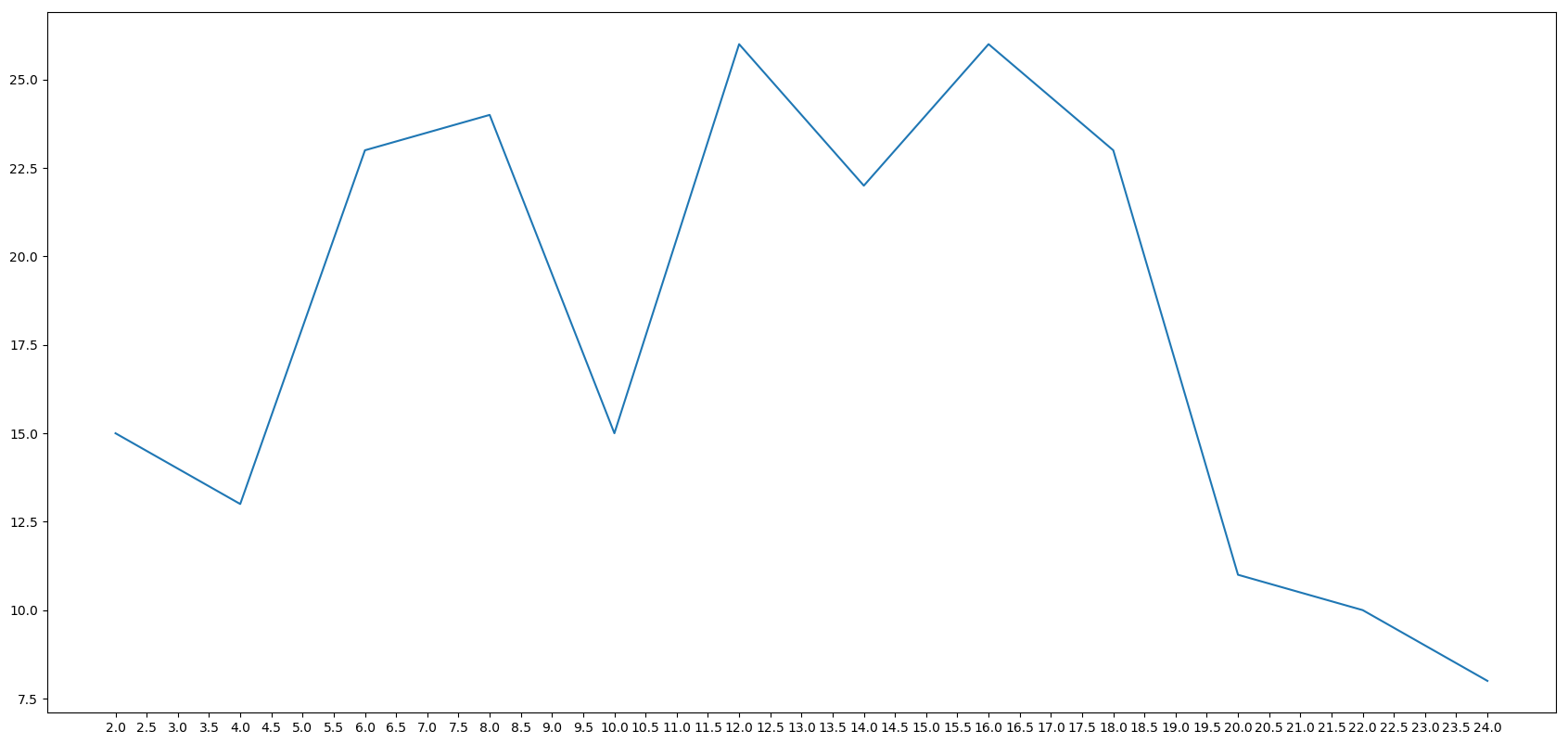y轴刻度

``````from matplotlib import pyplot as plt
x = range(2,26,2)
y = [15,13,23,24,15,26,22,26,23,11,10,8]#数据在y轴的位置，是一个可迭代对象
#->x轴和y轴的数据一起组成了所有要绘制出的坐标
#->分别是（2，15）（4，13）（6，23（8，24）......

plt.figure(figsize=(21,10),dpi=100)#设置图片大小
plt.plot(x,y)#->传入x和y，通过plot绘制出折线图
_stick_labels = [i/2 for i in range (4,49)]
plt.xticks(_stick_labels)#绘制x轴的刻度
plt.yticks(range(min(y),max(y)))
#plt.savefig("./sig_size.png")#保存图片，可以保存为svg这种矢量图格式，放大不会有据齿
plt.show()#->在执行程序的时候展示图形
``````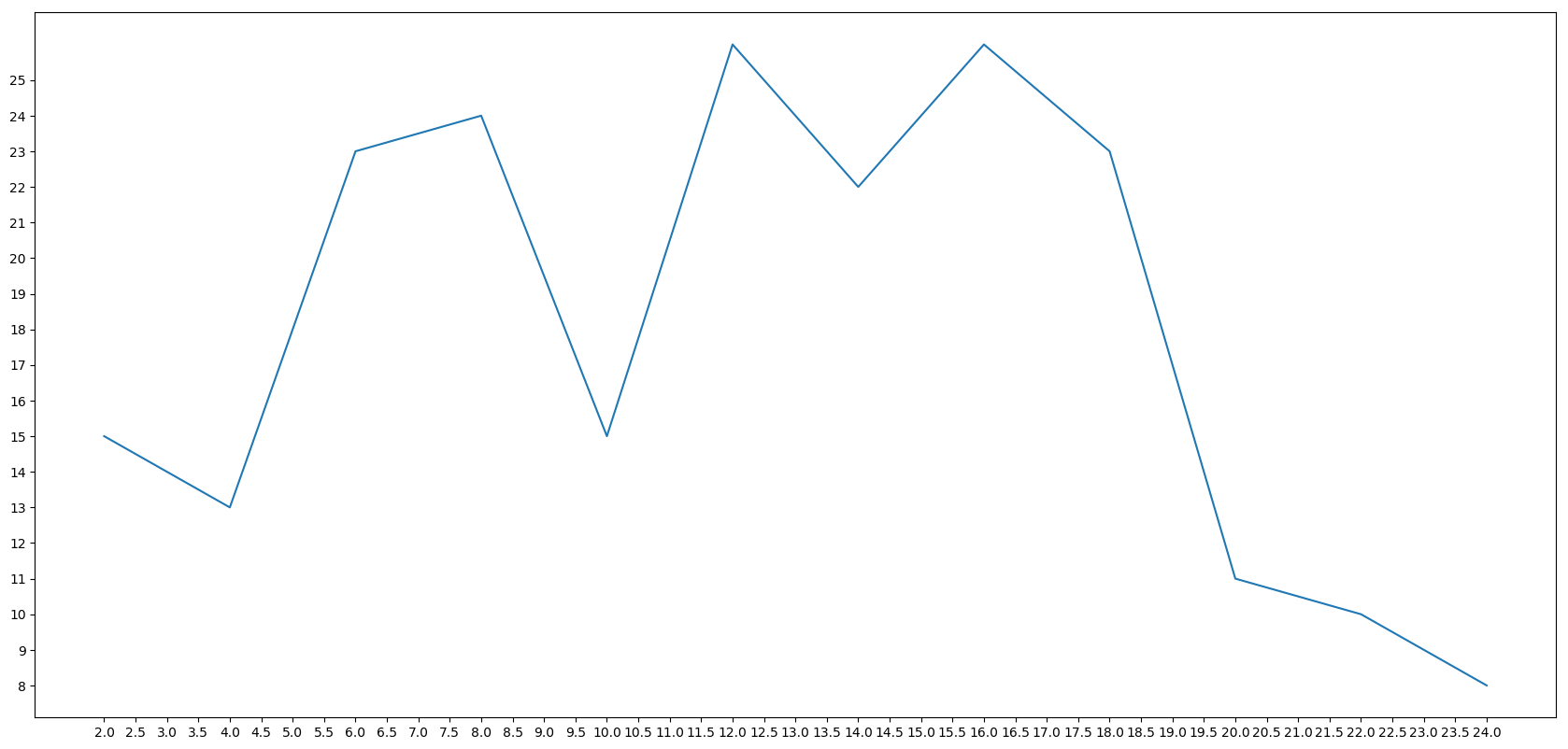a = [random.randint(23,37)for i in range(120)]

``````from matplotlib import pyplot as plt
import random
x = range(0,120)
y = [random.randint(23,37)for i in range(120)]
plt.plot(x,y)
plt.show()
``````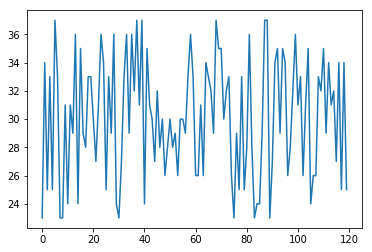``````from matplotlib import pyplot as plt
import random
x = range(0,120)
y = [random.randint(23,37)for i in range(120)]
plt.figure(figsize=(20,8),dpi=80)#设置图片大小
plt.plot(x,y)
_x = x
_xtick_labels = ["hello,{}".format(i) for i in _x]#调整x轴的刻度
plt.xticks(_x,_xtick_labels)
plt.show()
``````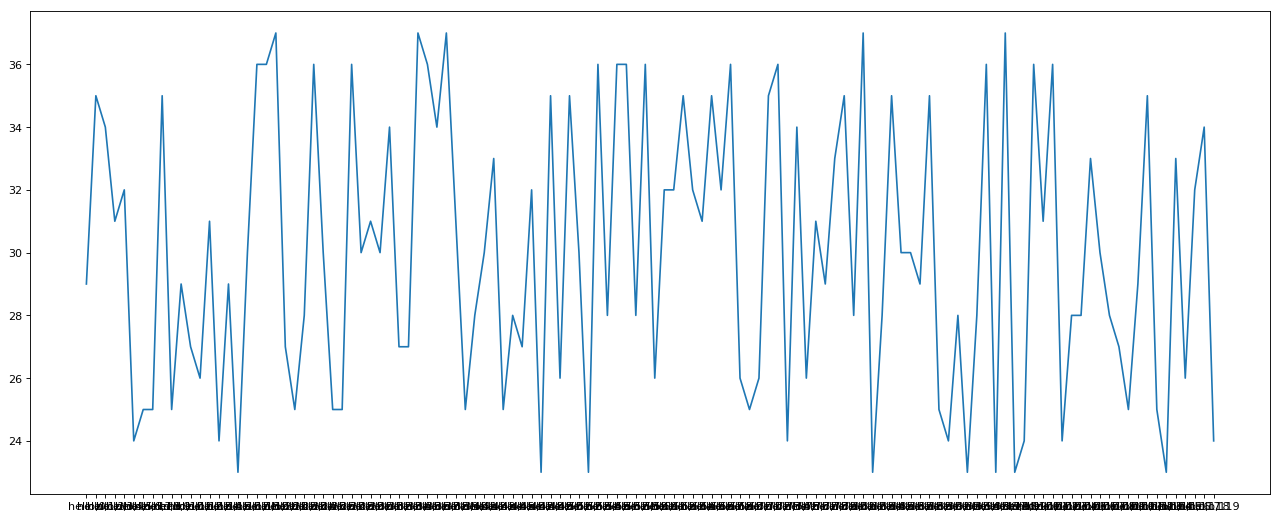# 调整x轴的刻度

_x = x
_xtick_labels = ["hello,{}".format(i) for i in _x]
plt.xticks(_x,_xtick_labels)

``````from matplotlib import pyplot as plt
import random
x = range(0,120)
y = [random.randint(23,37)for i in range(120)]
plt.figure(figsize=(20,8),dpi=80)
plt.plot(x,y)
_x = list(x)[::10]
_xtick_labels = ["hello,{}".format(i) for i in _x]#调整x轴的刻度
plt.xticks(_x,_xtick_labels)
plt.show()
``````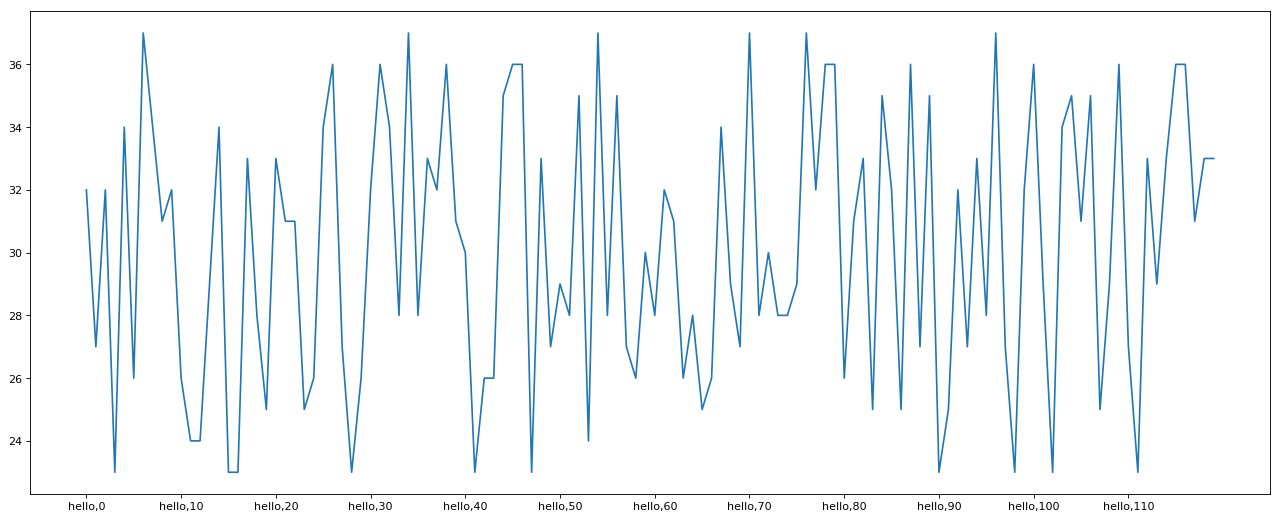``````from matplotlib import pyplot as plt
import random
x = range(0,120)
y = [random.randint(23,37)for i in range(120)]
plt.figure(figsize=(20,8),dpi=80)
plt.plot(x,y)
_xtick_labels = ["10点{}分".format(i) for i in range(60)]#调整x轴的刻度
_xtick_labels += ["11点{}分".format(i) for i in range(60)]
plt.xticks(list(x)[::3],_xtick_labels[::3])#取步长，数字和字符串一一对应，数据的长度一样
plt.show()
``````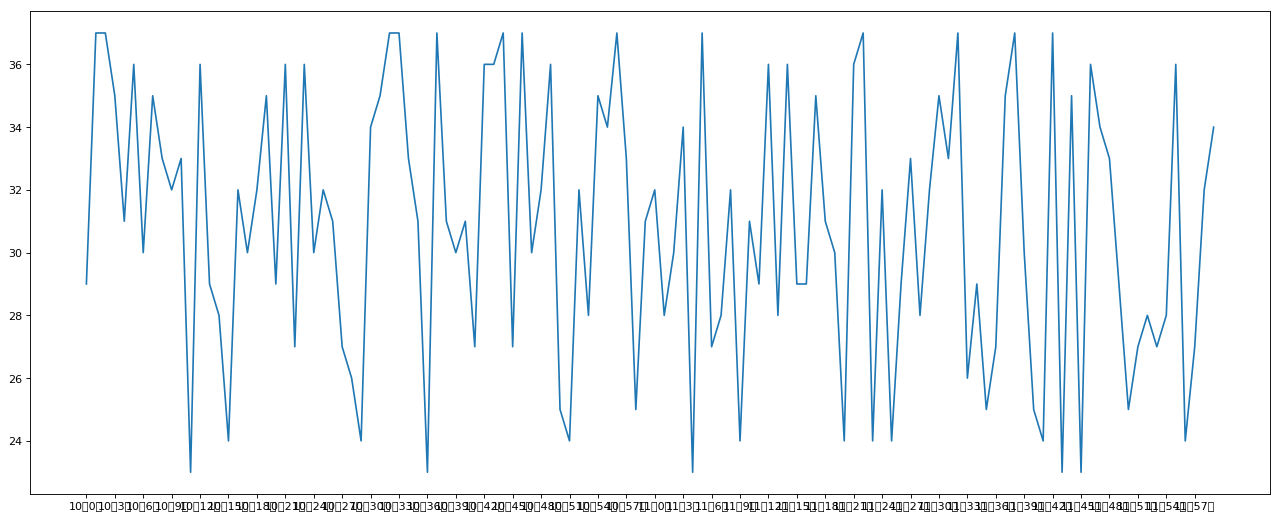``````from matplotlib import pyplot as plt
import random
x = range(0,120)
y = [random.randint(23,37)for i in range(120)]
plt.figure(figsize=(20,8),dpi=80)
plt.plot(x,y)
_xtick_labels = ["10点{}分".format(i) for i in range(60)]#调整x轴的刻度
_xtick_labels += ["11点{}分".format(i) for i in range(60)]
plt.xticks(list(x)[::3],_xtick_labels[::3],rotation=45)#取步长，数字和字符串一一对应，数据的长度一样
#rotation旋转的度数
plt.show()
``````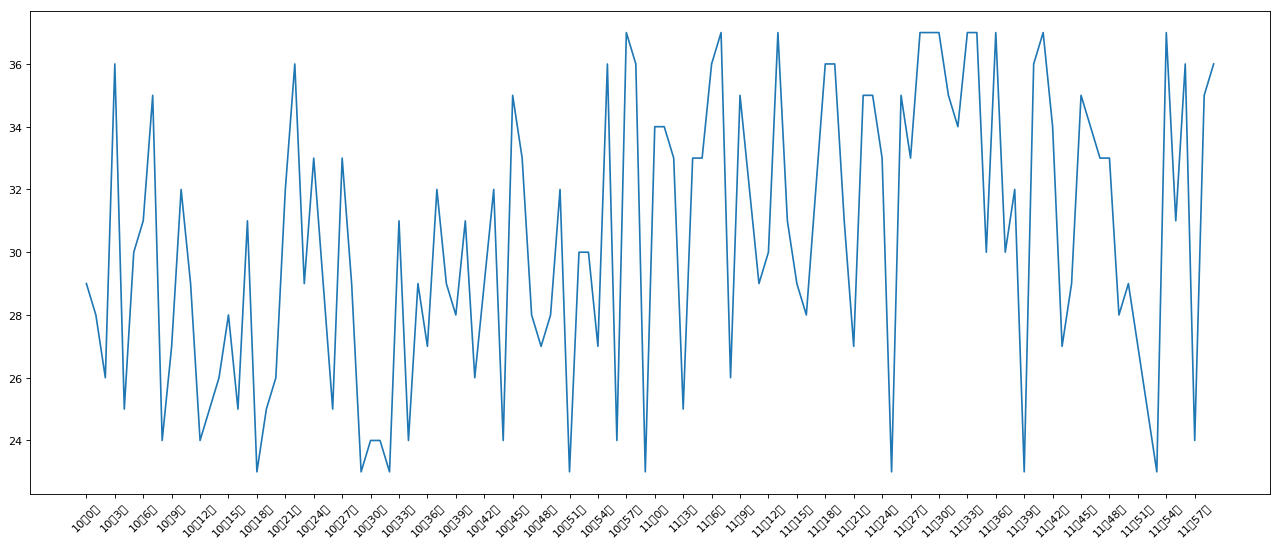matplotlib默认不支持中文字符，因为默认的英文字体无法显示汉字

fc-list->查看支持的字体

fc-list :lang=zh->查看支持的中文（冒号前面有空格）

``````from matplotlib import pyplot as plt
import random
import matplotlib
from matplotlib import font_manager
#windows&#x548C;linux&#x8BBE;&#x7F6E;&#x5B57;&#x4F53;&#x7684;&#x65B9;&#x5F0F;
#font = {'family':'Ubuntu-R.ttf',
#         'weight':'larger',
#         'size':'larger'}
#matplotlib.rc("font",**font)
#matplotlib.rc("font",famliy='Ubuntu-R.ttf','weight':'larger')
my_font = font_manager.FontProperties(fname="/static/fonts/Ubuntu-R.ttf")
x = range(0,120)
y = [random.randint(23,37)for i in range(120)]
plt.figure(figsize=(20,8),dpi=80)
plt.plot(x,y)
_xtick_labels = ["10&#x70B9;{}&#x5206;".format(i) for i in range(60)]#&#x8C03;&#x6574;x&#x8F74;&#x7684;&#x523B;&#x5EA6;
_xtick_labels += ["11&#x70B9;{}&#x5206;".format(i) for i in range(60)]
plt.xticks(list(x)[::3],_xtick_labels[::3],rotation=45,fontproperties=my_font)#&#x53D6;&#x6B65;&#x957F;&#xFF0C;&#x6570;&#x5B57;&#x548C;&#x5B57;&#x7B26;&#x4E32;&#x4E00;&#x4E00;&#x5BF9;&#x5E94;&#xFF0C;&#x6570;&#x636E;&#x7684;&#x957F;&#x5EA6;&#x4E00;&#x6837;
#rotation&#x65CB;&#x8F6C;&#x7684;&#x5EA6;&#x6570;
plt.show()
``````

Original: https://blog.csdn.net/qy_xm/article/details/122457481
Author: 旗妍
Title: python数据分析——numpy，pandas，matplotlib

# dirsearch安装+使用+运行问题（小宇特详解）

## 安装

dirsearch是一个用python开发的网站目录扫描工具
github下载地址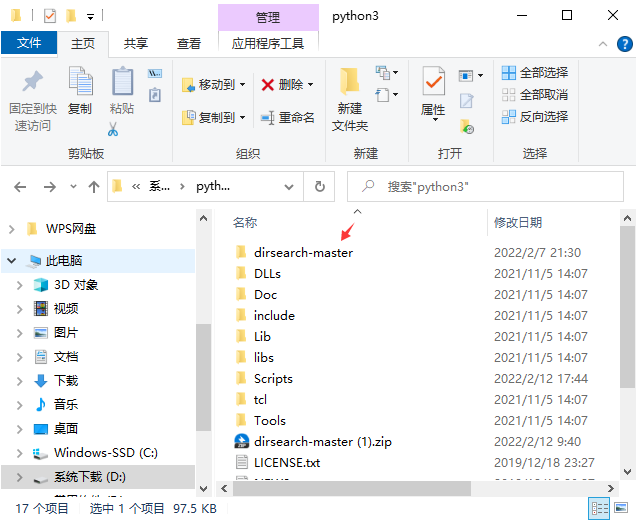## ; 使用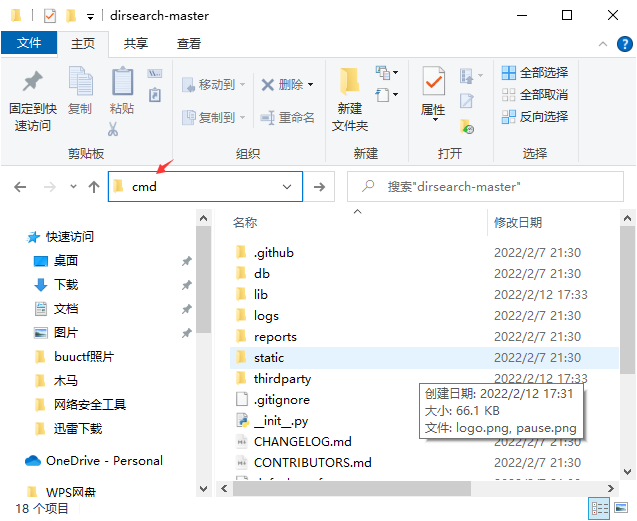``````python setup.py
``````

``````python dirsearch.py -u ip
``````

``````python dirsearch.py -h
``````

## 运行问题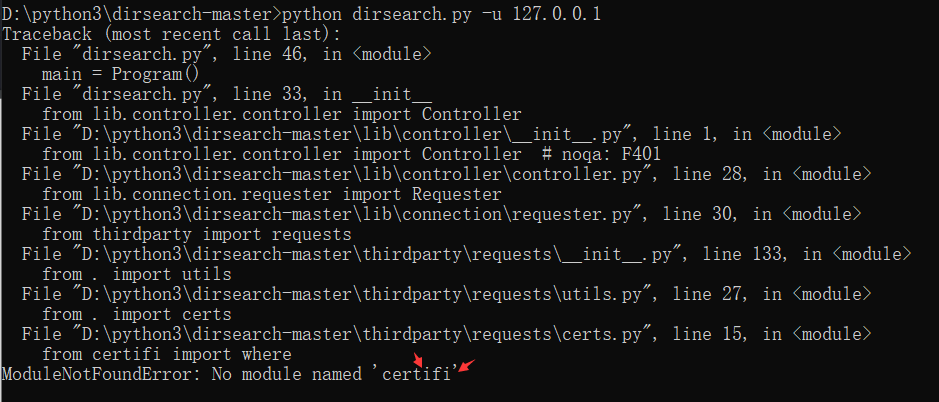``````pip install certifi
``````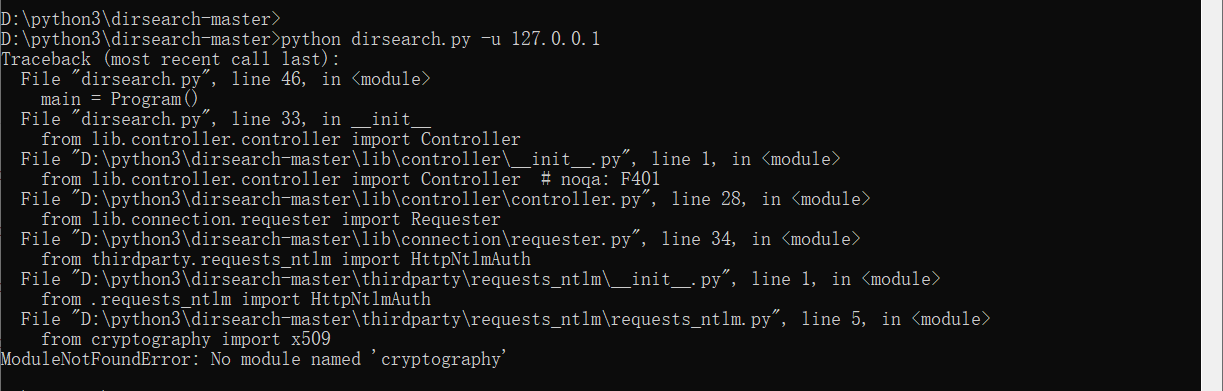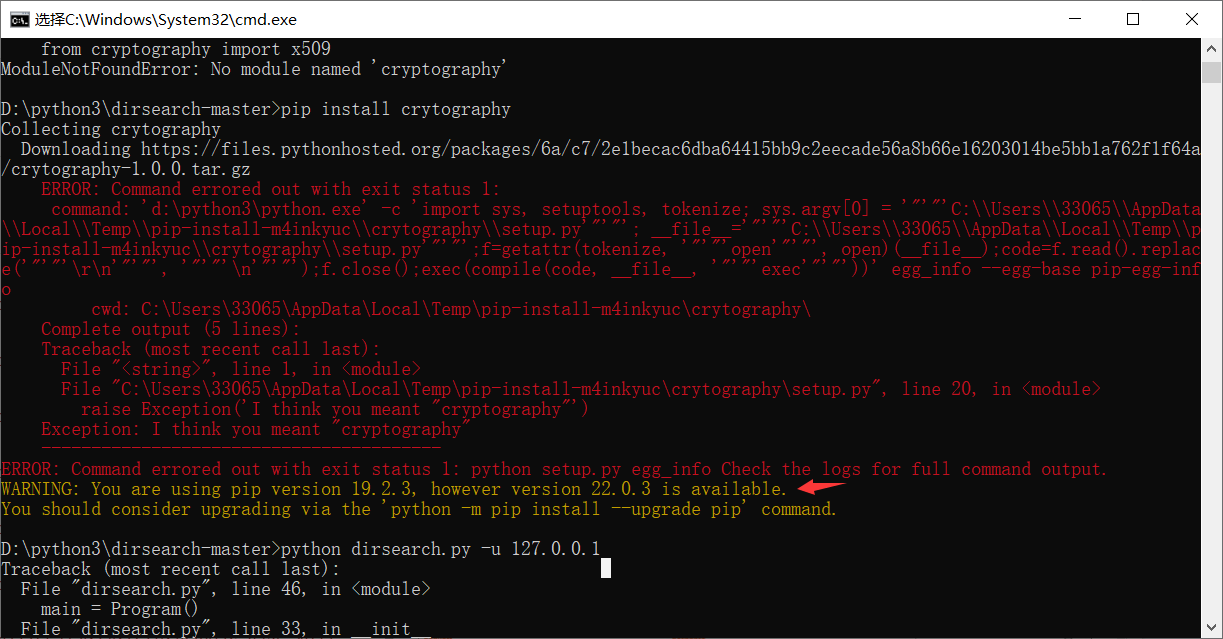``````pip -V
``````

``````D:\python3\python.exe -m pip install --upgrade pip
``````

``````pip install cryptography
``````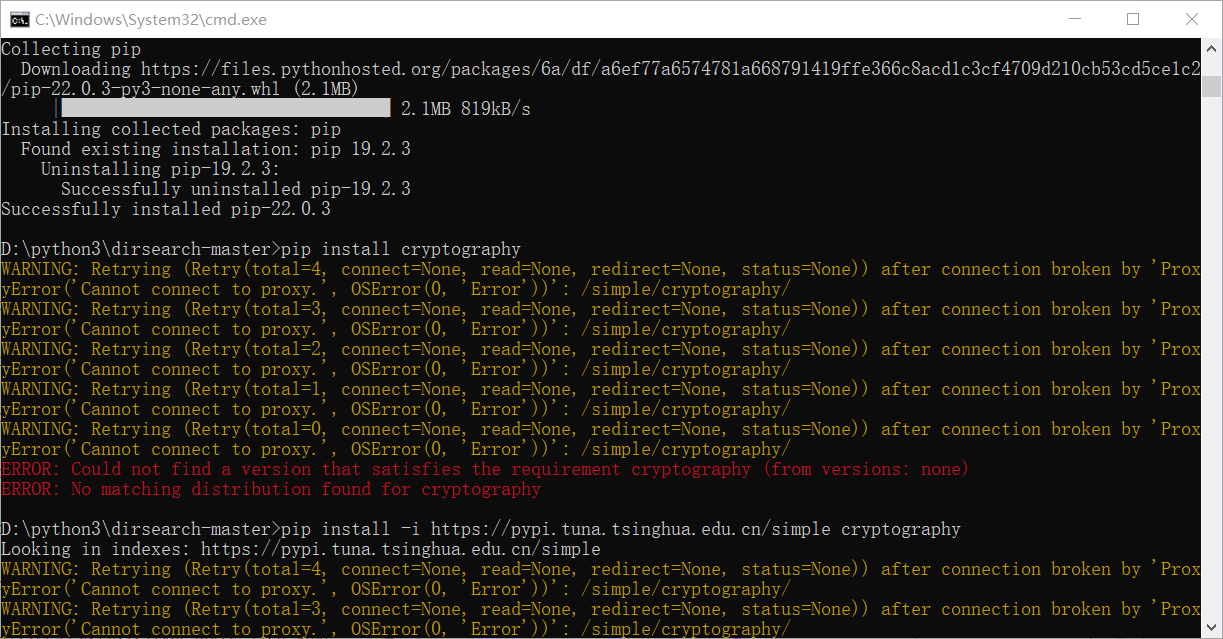### 希望我走过的坑你们不用再走。

Original: https://blog.csdn.net/xhy18634297976/article/details/122900500
Author: 小宇特详解
Title: dirsearch安装+使用+运行问题（小宇特详解）

## vmstat命令详解

vmstat命令是最常见的Linux/Unix监控工具，可以展现给定时间间隔的服务器的状态值,包括服务器的CPU使用率，内存使用，虚拟内存交换情况,IO读写情况。这个命令是我查看Linux/Unix最...